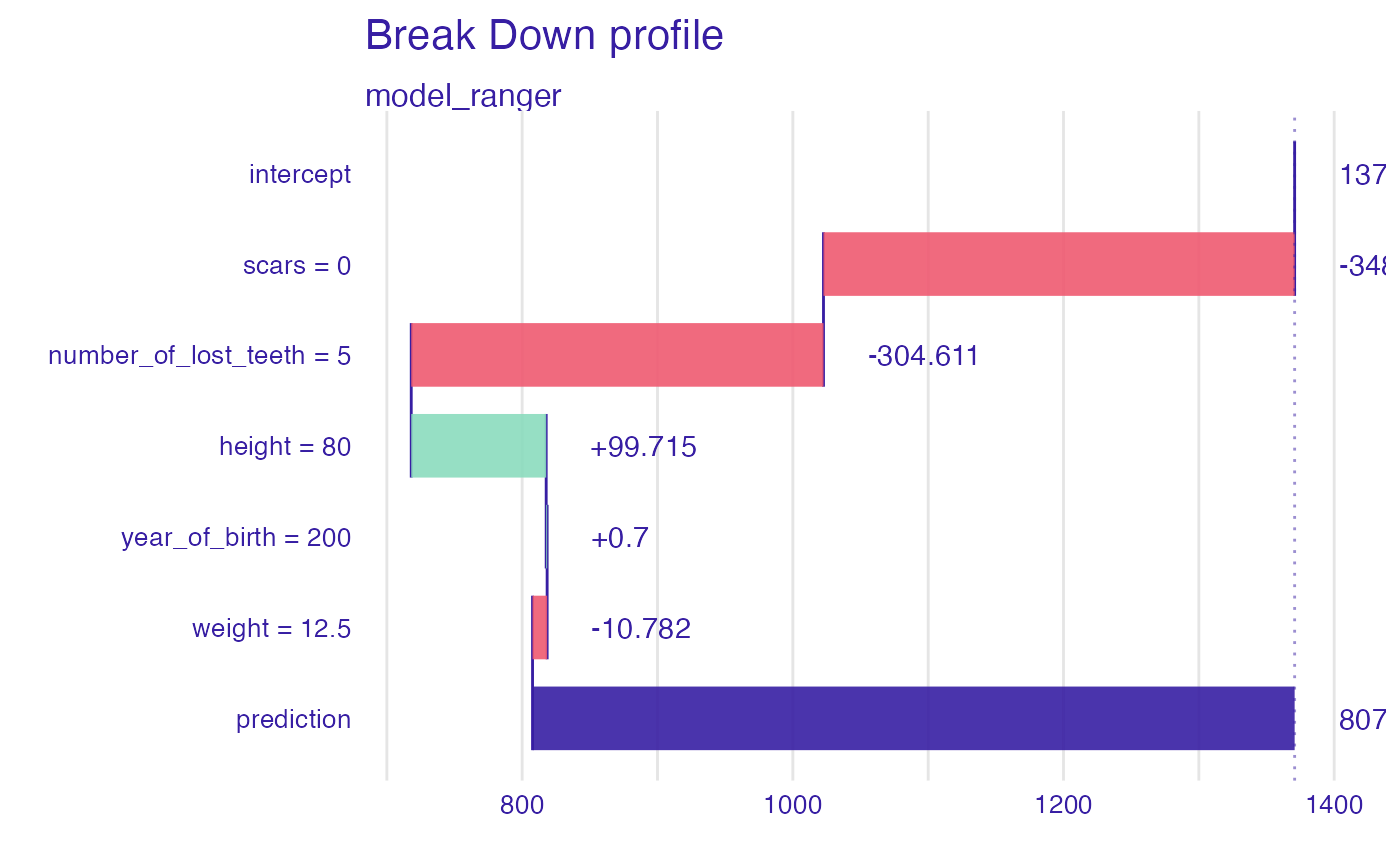Instance Level Variable Attributions as Break Down, SHAP, aggregated SHAP or Oscillations explanations. Model prediction is decomposed into parts that are attributed for particular variables. From DALEX version 1.0 this function calls the break_down or shap functions from the iBreakDown package or ceteris_paribus from the ingredients package or kernelshap from the kernelshap package. Find information how to use the break_down method here: https://ema.drwhy.ai/breakDown.html. Find information how to use the shap method here: https://ema.drwhy.ai/shapley.html. Find information how to use the oscillations method here: https://ema.drwhy.ai/ceterisParibusOscillations.html. Find information how to use the kernelshap method here: https://modeloriented.github.io/kernelshap/ aSHAP method provides explanations for a set of observations based on SHAP.

predict_parts(
explainer,
new_observation,
...,
N = if (substr(type, 1, 4) == "osci") 500 else NULL,
type = "break_down"
)

predict_parts_oscillations(explainer, new_observation, ...)

predict_parts_oscillations_uni(
explainer,
new_observation,
variable_splits_type = "uniform",
...
)

predict_parts_oscillations_emp(
explainer,
new_observation,
variable_splits = NULL,
variables = colnames(explainer$data), ... ) predict_parts_break_down(explainer, new_observation, ...) predict_parts_break_down_interactions(explainer, new_observation, ...) predict_parts_shap(explainer, new_observation, ...) predict_parts_shap_aggregated(explainer, new_observation, ...) predict_parts_kernel_shap(explainer, new_observation, ...) predict_parts_kernel_shap_break_down(explainer, new_observation, ...) predict_parts_kernel_shap_aggreagted(explainer, new_observation, ...) variable_attribution( explainer, new_observation, ..., N = if (substr(type, 1, 4) == "osci") 500 else NULL, type = "break_down" ) ## Arguments explainer a model to be explained, preprocessed by the explain function new_observation a new observation for which predictions need to be explained ... other parameters that will be passed to iBreakDown::break_down N the maximum number of observations used for calculation of attributions. By default NULL (use all) or 500 (for oscillations). type the type of variable attributions. Either shap, aggregated_shap, oscillations, oscillations_uni, oscillations_emp, break_down, break_down_interactions, kernel_shap, kernel_shap_break_down or kernel_shap_aggregated. variable_splits_type how variable grids shall be calculated? Will be passed to ceteris_paribus. variable_splits named list of splits for variables. It is used by oscillations based measures. Will be passed to ceteris_paribus. variables names of variables for which splits shall be calculated. Will be passed to ceteris_paribus. ## Value Depending on the type there are different classes of the resulting object. It's a data frame with calculated average response. ## Examples library(DALEX) new_dragon <- data.frame( year_of_birth = 200, height = 80, weight = 12.5, scars = 0, number_of_lost_teeth = 5 ) model_lm <- lm(life_length ~ year_of_birth + height + weight + scars + number_of_lost_teeth, data = dragons) explainer_lm <- explain(model_lm, data = dragons, y = dragons$year_of_birth,
label = "model_lm")
#> Preparation of a new explainer is initiated
#>   -> model label       :  model_lm
#>   -> data              :  2000  rows  8  cols
#>   -> target variable   :  2000  values
#>   -> predict function  :  yhat.lm  will be used (  default  )
#>   -> predicted values  :  No value for predict function target column. (  default  )
#>   -> model_info        :  package stats , ver. 4.2.3 , task regression (  default  )
#>   -> predicted values  :  numerical, min =  541.1056 , mean =  1370.986 , max =  3928.189
#>   -> residual function :  difference between y and yhat (  default  )
#>   -> residuals         :  numerical, min =  -5421.316 , mean =  -1450.523 , max =  1176.912
#>   A new explainer has been created!

bd_lm <- predict_parts_break_down(explainer_lm, new_observation = new_dragon)
#>                                    contribution
#> model_lm: intercept                    1370.986
#> model_lm: scars = 0                    -397.300
#> model_lm: number_of_lost_teeth = 5     -302.277
#> model_lm: height = 80                    -9.734
#> model_lm: year_of_birth = 200            -6.639
#> model_lm: weight = 12.5                  -0.973
plot(bd_lm)# \donttest{
library("ranger")
model_ranger <- ranger(life_length ~ year_of_birth + height +
weight + scars + number_of_lost_teeth,
data = dragons, num.trees = 50)

explainer_ranger <- explain(model_ranger,
data = dragons,
y = dragons\$year_of_birth,
label = "model_ranger")
#> Preparation of a new explainer is initiated
#>   -> model label       :  model_ranger
#>   -> data              :  2000  rows  8  cols
#>   -> target variable   :  2000  values
#>   -> predict function  :  yhat.ranger  will be used (  default  )
#>   -> predicted values  :  No value for predict function target column. (  default  )
#>   -> model_info        :  package ranger , ver. 0.14.1 , task regression (  default  )
#>   -> predicted values  :  numerical, min =  585.2637 , mean =  1370.777 , max =  3390.243
#>   -> residual function :  difference between y and yhat (  default  )
#>   -> residuals         :  numerical, min =  -4961.243 , mean =  -1450.314 , max =  1052.711
#>   A new explainer has been created!

bd_ranger <- predict_parts_break_down(explainer_ranger, new_observation = new_dragon)